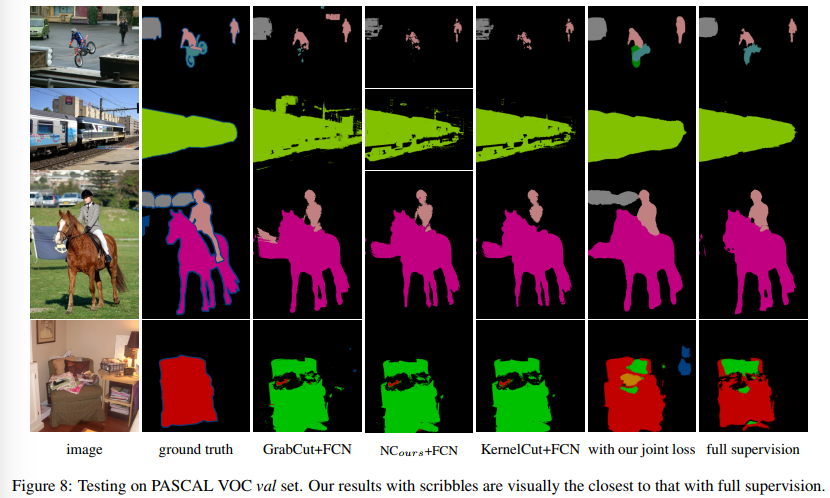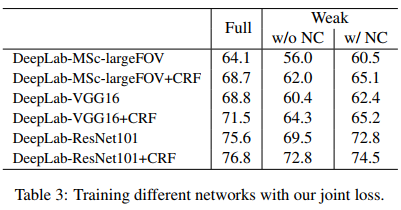## Introduction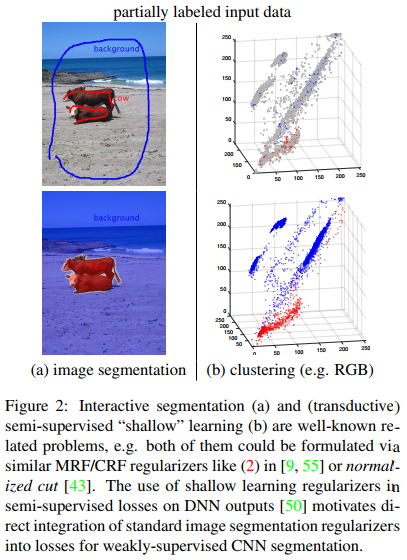They proposed a weakly-supervised approach to generate full masks from partial inputs (e.g. scribbles or seeds) using standard interactive segmentation methods as preprocessing. To ensure a more accurate result, they propose a new loss function inspired by a well-known criteria used in unsupervised segmentation, e.g. normalized cut.

## Method

Normalized cut is a method that comes from the spectral clustering theory. In short, for a 2-class segmentation problem, given a graph $$\Omega$$, it looks for two complementary subgraphs $$\Omega_k$$ and $$\Omega/\Omega_k$$ that would minimizes the following loss: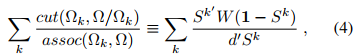Where $$cut$$ is the cost of cutting the graph in two and $$assoc$$ is the size of the two subgraphs. This energy term can be reformulated as the element on the right hand side of (4), i.e. $$S^k$$ a binary indicator vector for subgraph $$\Omega_k$$, $$W$$ is a nb_pixels x nb_pixels similarity matrix, $$d$$ is a degree vector : $$d_i =\sum_j W_{ij}$$.

Since the minimization of (4) is NP-Hard, as usual $$S^k$$ is replaced by a soft vector with values between 0 and 1.

The proposed loss is the sum of a usual cross-entropy and a normalized cut term: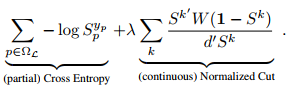They also show that this Normalized cut is differentiable and thus end-to-end trainable.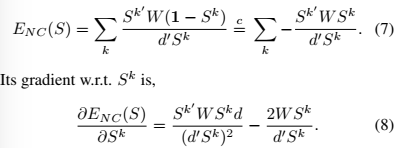## Results

Results of their method are close to that obtained with full supervision